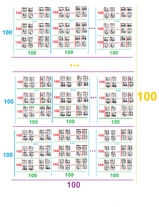11,051 Pages

The Heptfactor is equal to 100!7 using Extensible ! Notation. The term was coined by Yabuszko. This number is comparable to gagol and gaspgol.Visual representation of heptfactor

## Etymology

The name of this number is based on Greek prefix "hepta-" and the factor.

## Approximations

Notation Approximation
Arrow notation $$82\uparrow^7101$$
Chained Arrow Notation $$82 \rightarrow 101 \rightarrow 7$$
BEAF $$\{82,101,7\}$$
Hyper-E notation

$$E160\#100\#\#6$$

Hyperfactorial Array Notation $$103!6$$
Strong array notation $$s(82,101,7)$$
Nested Factorial Notation $$100!$$
Fast-growing hierarchy $$f_8(100)$$
Hardy hierarchy $$H_{\omega^8}(100)$$
Slow-growing hierarchy $$g_{\varphi(6,0)}(100)$$

## Sources

Community content is available under CC-BY-SA unless otherwise noted.